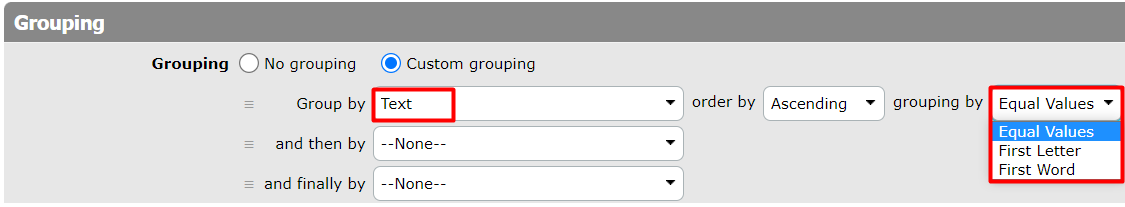# Grouping

There is the `Grouping` section listed among view properties (except Calendar View), where you can specify the way how the displayed records will be grouped.

Grouping is quite simple and obvious; moreover, there are some additional options specifying the grouping. Such options depend on the type of column used for grouping.

If you select the Date or Formula-Date column, you can group by:

Equal Values, Day, Week, Month, Quarter, Year, Day in Month, Month in Year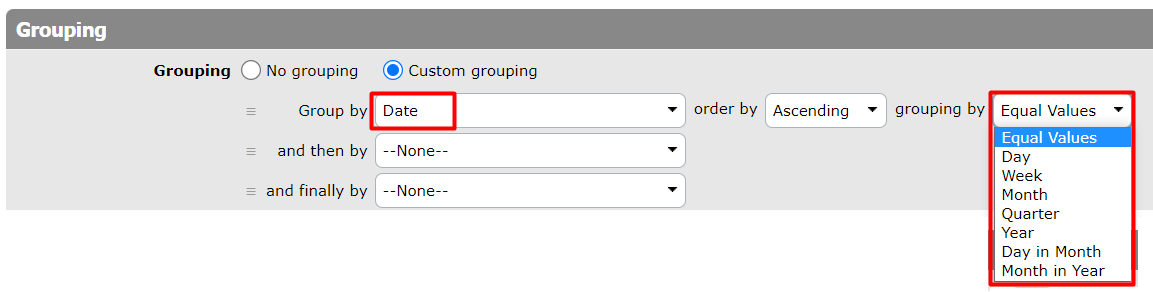If you choose the Time Column or Formula-Time, you can group by:

Equal Values, Second, Minute, Hour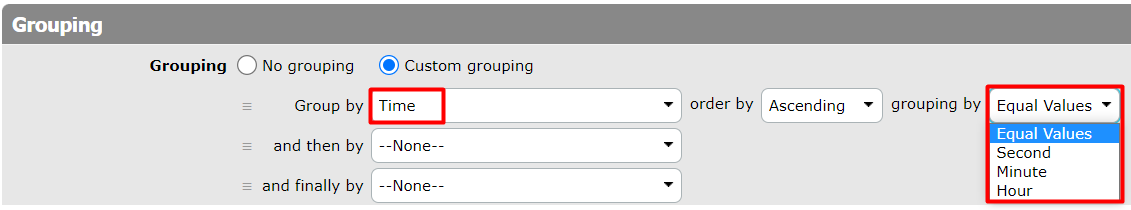If you choose the Timestamp or Formula-Timestamp Column, you can group by:

Equal Values, Second, Minute, Hour, Day, Week, Month, Quarter, Year, Day in Month, Month in Year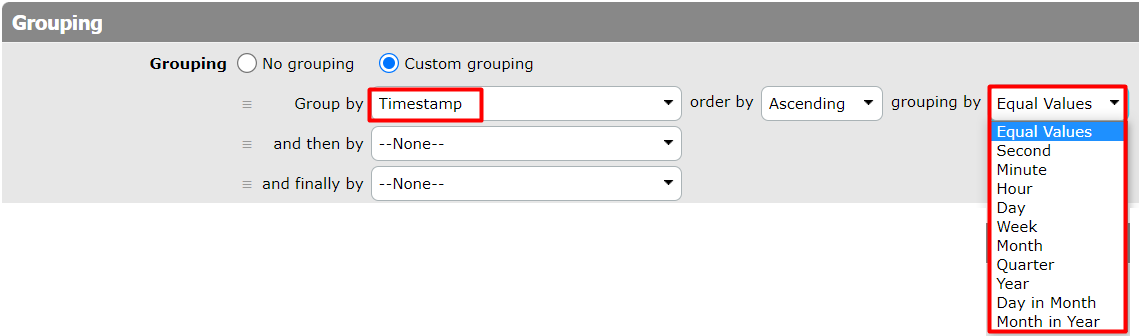If you choose the Duration Column or Formula-Duration, you can group by:

Equal Values, Second, Minute, Hour, Day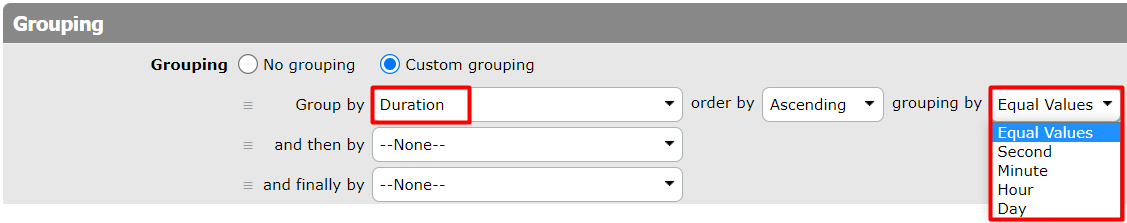If you choose the Numeric Column or Formula-Numeric, you can group by: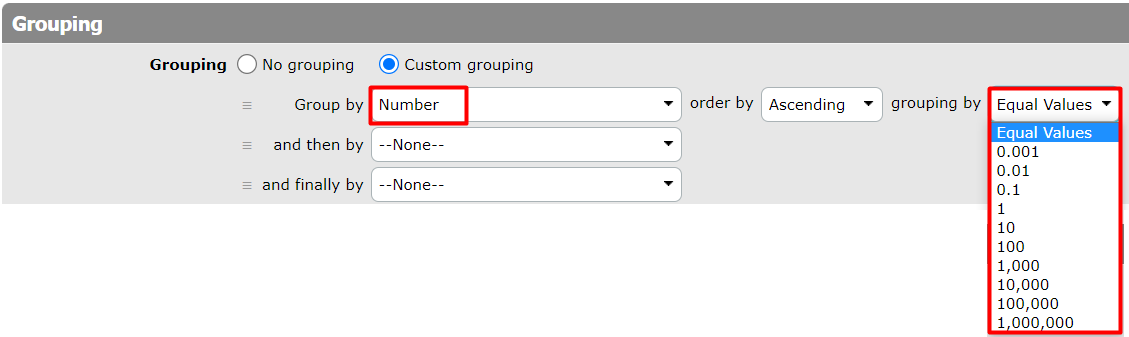If you choose the Text Column or Formula-Text, you can group by:

Equal Values, First Letter, First Word